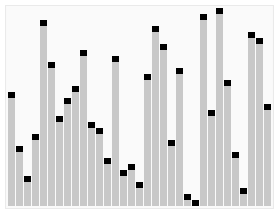# Quicksort• Divide and conquer
• Divides a large list into smaller sub-lists
• Low elements and high elements
• Recursively sorts the sub-lists
• Usually done in place with $O(log(n))$ stack space

## Complexity

Average TimeSpace
$O(n log(n))$$log(n)$

## Process

• Pick an element to be the pivot point
• Partition
• Reorder list
• All elements with value less than pivot come before the pivot
• All elements with value greater than pivot come after the pivot
• Pivot is in its final position
• Recursively apply above steps to sub-list of elements with smaller values and greater values

## Implementation

### JavaScript

function quickSort(array, lowIndex = 0, highIndex = array.length - 1) {
// partitionArray operates on the subarray between lowIndex and highIndex (inclusive)
// Partially sorts subarray into elements less than pivot and elements greater than or equal to pivot
// Each time it is executed, pivot element is in iits final sorted position
const partitionArray = (lowIndex, highIndex) => {
// Swap two elements
const swap = (leftIndex, rightIndex) => {
const temp = array[leftIndex];
array[leftIndex] = array[rightIndex];
array[rightIndex] = temp;
}

// Arbitraily pick last element as a pivot point
const pivot = array[highIndex];

let partitionIndex = lowIndex;
for (let currIndex = lowIndex; currIndex < highIndex; currIndex++) {
if (array[currIndex] < pivot) {
swap(partitionIndex, currIndex);
partitionIndex++;
}
}

// Element at partitionIndex must be greater than or equal to pivot
// Elements to the left are less than pivot
// Swapping pivot with partitionIndex places pivot in final sorted position
swap(partitionIndex, highIndex);

return partitionIndex;
}

if (lowIndex < highIndex) {
const partitionIndex = partitionArray(lowIndex, highIndex);
quickSort(array, lowIndex, partitionIndex - 1);
quickSort(array, partitionIndex + 1, highIndex);
}

return array;
}